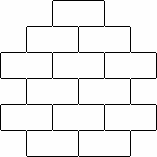ENG  RUS Timus Online JudgeOnline Judge
Problems
Authors
Online contests
Site news
Webboard
Problem set
Submit solution
Judge status
Guide
Register
Authors ranklist
Current contest
Scheduled contests
Past contests
Rules

Contest is over

## G. Building Towers

Time limit: 1.0 second
Memory limit: 4 MB
There are N bricks in a toy box which have 1-unit height and 2-unit width. The teacher organizes a tower-building game. The tower is built of the bricks. The tower consists of H levels. The bottom level contains M bricks, every next level must contain exactly one brick less or greater than the level just below it.
Here is an example of a tower with H=6, M=2, N=13.The tower with H levels can be represented by the array of H integers, which are the numbers of bricks in each level from the bottom to the top. Consider all different towers with exactly H levels and exactly M bricks in the bottom level that can be built using not more than N bricks. We can number these towers in such way that corresponding arrays will be ordered lexicographically.
Your task is to find a tower with specific number in the sense of described order.

### Input

The first line of the input contains positive numbers N, H and M (N ≤ 32767, H ≤ 60, M ≤ 10). Each of the following lines contains integer K, which is the interested tower number. The last line contains number -1. The towers are numbered starting from 1.

### Output

The first line of the output should contain the total number of different towers that can be built. Each following line should contain an array describing the tower with number K given in respective line of input. The numbers in the arrays should be separated by at least one space.

### Sample

inputoutput
```22 5 4
1
10
-1
```
```10
4 3 2 1 2
4 5 4 5 4
```
To submit the solution for this problem go to the Problem set: 1148. Building Towers